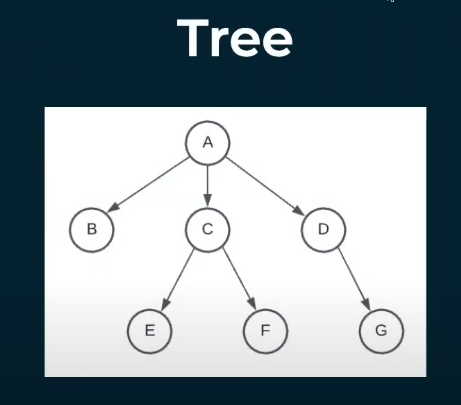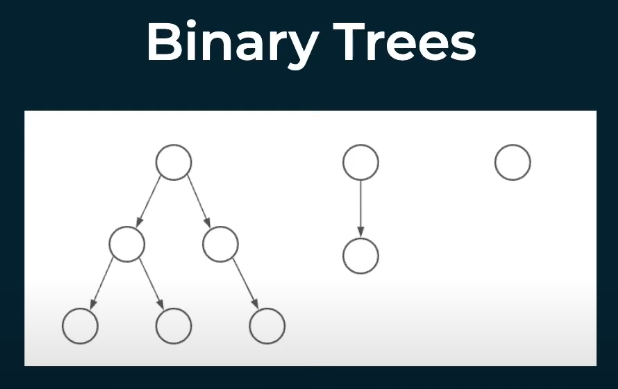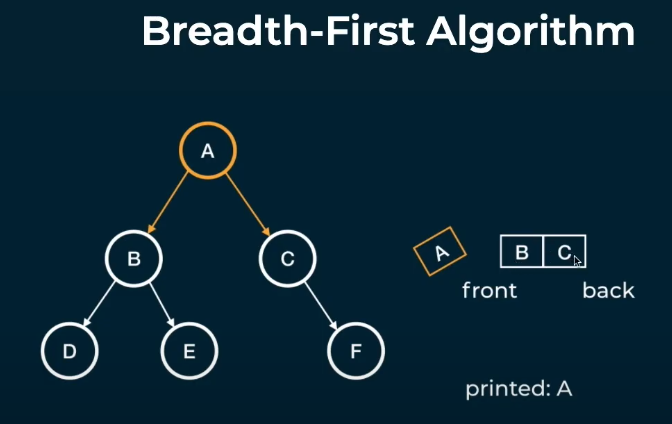# 6.Binary Trees and BFS

kimkevin90·2021년 11월 26일
0

## 1. Whats's a tree?A collection of nodeds and edges

• 하나의 루트 노드
• 노드로 가기 위한 길은 오직 하나

## 2. Whats's Binary tree?Tree where each node has at most two children

• 최대 노드 수 2개, 한개거나 없어도 binary tree라 볼 수 있다.

코드 구현

class Node {
constructor(val) {
this.val = val;
this.left = null;
this.right = null;
}
}

const a = new Node('a');
const b = new Node('b');
const c = new Node('c');
const d = new Node('d');
const e = new Node('e');
const f = new Node('f');

a.left = b;
a.right = c;
b.left = d;
b.right = e;
c.right = f;Starting at the root and Exploring all nodes in the same level

• 해당 계층의 모든 노드를 탐색 후 아래 계층 탐색• Using Queue
• 큐스택에 쌓인 노드를 dequeue, 자식 노드가 있다면 자식 노드를 enqueue

코드 구현

class Node {
constructor(val) {
this.val = val;
this.left = null;
this.right = null;
}
}

const a = new Node('a');
const b = new Node('b');
const c = new Node('c');
const d = new Node('d');
const e = new Node('e');
const f = new Node('f');

a.left = b;
a.right = c;
b.left = d;
b.right = e;
c.right = f;

const breadthFirstPrint = (root) => {
const queue = [ root ];
while (queue.length > 0) {
const curr = queue.shift();
console.log(curr.val);
if(curr.left !== null) {
queue.push(curr.left);
}

if(curr.right !== null) {
queue.push(curr.right);
}
}
};

const bfs = (root, target) => {
const queue = [ root ];
while (queue.length > 0) {
const curr = queue.shift();
if(curr.val === target) {
return true;
}

if(curr.left !== null) {
queue.push(curr.left);
}

if(curr.right !== null) {
queue.push(curr.right);
}
}

return false;
};

const sumTree = (root) => {
const queue = [ root ];
let sum = 0;
while (queue.length > 0) {
const curr = queue.shift();
sum += curr.val;
if(curr.left !== null) {
queue.push(curr.left);
}

if(curr.right !== null) {
queue.push(curr.right);
}
}

return sum;
};

console.log(bfs(a, "z")); // false